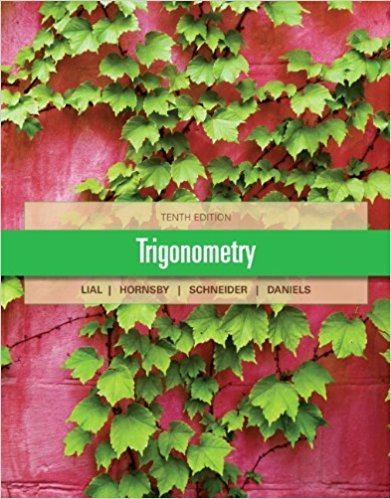×

×

# Solutions for Chapter 7.1 - 7.3: Quiz## Full solutions for Trigonometry | 10th Edition

ISBN: 9780321671776Solutions for Chapter 7.1 - 7.3: Quiz

Solutions for Chapter 7.1 - 7.3
4 5 0 405 Reviews
14
0
##### ISBN: 9780321671776

Chapter 7.1 - 7.3: Quiz includes 10 full step-by-step solutions. Trigonometry was written by and is associated to the ISBN: 9780321671776. Since 10 problems in chapter 7.1 - 7.3: Quiz have been answered, more than 35604 students have viewed full step-by-step solutions from this chapter. This textbook survival guide was created for the textbook: Trigonometry, edition: 10. This expansive textbook survival guide covers the following chapters and their solutions.

Key Math Terms and definitions covered in this textbook
• Cholesky factorization

A = CTC = (L.J]))(L.J]))T for positive definite A.

• Circulant matrix C.

Constant diagonals wrap around as in cyclic shift S. Every circulant is Col + CIS + ... + Cn_lSn - l . Cx = convolution c * x. Eigenvectors in F.

• Column space C (A) =

space of all combinations of the columns of A.

• Complex conjugate

z = a - ib for any complex number z = a + ib. Then zz = Iz12.

A sequence of steps (end of Chapter 9) to solve positive definite Ax = b by minimizing !x T Ax - x Tb over growing Krylov subspaces.

• Cross product u xv in R3:

Vector perpendicular to u and v, length Ilullllvlll sin el = area of parallelogram, u x v = "determinant" of [i j k; UI U2 U3; VI V2 V3].

• Cyclic shift

S. Permutation with S21 = 1, S32 = 1, ... , finally SIn = 1. Its eigenvalues are the nth roots e2lrik/n of 1; eigenvectors are columns of the Fourier matrix F.

• Echelon matrix U.

The first nonzero entry (the pivot) in each row comes in a later column than the pivot in the previous row. All zero rows come last.

• Fundamental Theorem.

The nullspace N (A) and row space C (AT) are orthogonal complements in Rn(perpendicular from Ax = 0 with dimensions rand n - r). Applied to AT, the column space C(A) is the orthogonal complement of N(AT) in Rm.

• Length II x II.

Square root of x T x (Pythagoras in n dimensions).

• Linear combination cv + d w or L C jV j.

• Orthonormal vectors q 1 , ... , q n·

Dot products are q T q j = 0 if i =1= j and q T q i = 1. The matrix Q with these orthonormal columns has Q T Q = I. If m = n then Q T = Q -1 and q 1 ' ... , q n is an orthonormal basis for Rn : every v = L (v T q j )q j •

• Outer product uv T

= column times row = rank one matrix.

• Pivot.

The diagonal entry (first nonzero) at the time when a row is used in elimination.

• Rank r (A)

= number of pivots = dimension of column space = dimension of row space.

• Right inverse A+.

If A has full row rank m, then A+ = AT(AAT)-l has AA+ = 1m.

• Row space C (AT) = all combinations of rows of A.

Column vectors by convention.

• Spectral Theorem A = QAQT.

Real symmetric A has real A'S and orthonormal q's.

• Spectrum of A = the set of eigenvalues {A I, ... , An}.

Spectral radius = max of IAi I.

• Toeplitz matrix.

Constant down each diagonal = time-invariant (shift-invariant) filter.